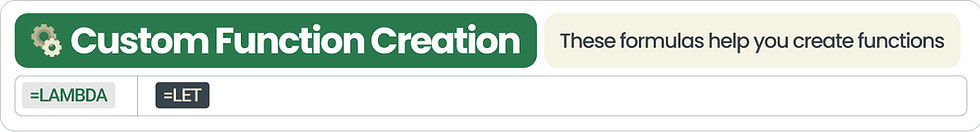top of page

# Excel Formulas Cheat Sheet## There are an endless amount of formulas in excel...

but knowing how to use each, as well as when to use each is what separates the pros from the beginners.

Let's do a deep dive into all of our options so that by the end of today, you'll be able to call yourself a pro 😎

### ➡️Sum Formulas

These are fairly simple - they allow you to sum values in a range### ➡️Lookup Formulas

These are really common → they return a value that you enter into your lookup formula

*Note: there's no reason to use vlookup anymore!### ➡️ Date Formulas

These are formulas that allow you to work flexibly with dates ​

My favorite here is EOMONTH### ➡️ ROUND Formulas### ➡️ CONDITIONAL / BOOLEAN Formulas

These are also really popular, and allow you to return results that meet a specific true / false criterion### ➡️ SPILL ARRAY Formulas

These formulas #SPILL! into other cells.

These are really useful, and are unique in the way they feed into other cells### ➡️General Math Formulas

These formulas are basic math operators, but make no mistake...they can be really powerful.### ➡️Text Formulas

These excel formulas are helpful when dealing with text.

Trim is especially helpful when working with accounting softwares that add a space before / after your GL account### ➡️Finance Formulas

These excel formulas are useful in the context of finance.

I'll often times use one of these when building out a debt schedule.### ➡️Custom Function Creation Formula

I LOVE using LET, as it helps me create variables that I can reuse in my formulas### ➡️Indirect Cell/Range Reference Formula

These excel formulas allow you to refer to cells, both directly and indirectly.

I like to sometimes create a reference to a tab in a cell, and use that text in my formulas using indirect.### ➡️Combine Formulas

These excel formulas allow you to combine values### ➡️10 Formulas that I use EVERYDAY in Finance & Accounting from the Excel Cheat Sheet

In my job, I am constantly using excel...

and when I go on vacation, I have withdrawal 🤣

#### Here are the formulas I use everyday from the excel cheat sheet:

• EOMONTH

• SUMIFS

• INDEX / MATCH

• COUNTIFS

• SUMPRODUCT

• LET

• FILTER

• UNIQUE

• SORT

• IF

Here's the full Excel Formulas Cheat Sheet

573 views

See All
bottom of page